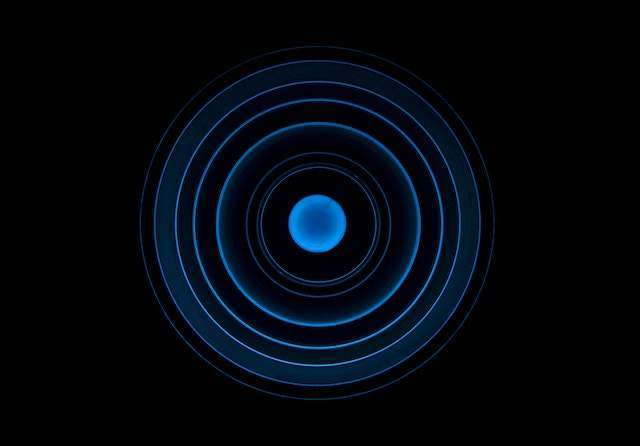# Diameter to Circumference Calculator

Instructions: Use this calculator to compute the circumference of a circle with a given diameter you provide, showing all the steps. Please type the diameter d in the box below.Enter the diameter d. It must be positive (Ex: 2, or 3/4, etc.)

This calculator will allow you to compute the circumference of a circle directly from its diameter, showing all the steps of the process. All you need to provide is a valid numeric expression for the diameter. It could be a number or fraction, or even a compound numeric expression, provided that it is greater than 0.

Once you provide a valid diameter d, you just need to click on the "Calculate" button, and all the steps of the process will be shown and presented to you .

Also, you may be interested in the reverse process, which is how to calculate the diameter from the circumferencefrom a circle.## Diameter to Circumference Formula Calculation

The most typical situation is to get the circumference of a circle starting with the radius, but there is a shortcut in the formula that allows you to go directly from diameter to circumference, as shown in the formula below:

$C = \pi d$

Could it get any easier? You just multiply the diameter d by $$\pi$$.

## What are the steps for going from diameter to circumference?

• Step 1: Identify the diameter d and its potential unit length. It needs to be positive, otherwise you cannot proceed
• Step 2: Once you have a valid diameter d, the circumference is obtained by multiplying d by π
• Step 3: After computing C = π d, you leave the answer in terms of π, or evaluate numerically.

In the most typical case, the circumference result will depend on π, so you may want to evaluate the expression to get a numeric value.

Perhaps, you are the kind of chap that don't like diameters and prefers working with a radius, in which case you will remember that d = 2r, so then, you can compute the radius from the diameter as shown below:

$\displaystyle r = \frac{d}{2}$

In layman terms, the radius is half of diameter

## What is the circumference of 12 inch diameter?

This is an example that can be used to understand the formula. So, the diameter is directly provided as d = 12 inch, and it comes with a length unit.

From the formula shown above, the circumference is C = π d = 12 π inches. Now, if we wanted to convert this to its numerical value we get that C = 37.699112 inches.

## Why would I use the diameter to compute the circumference?

Good point. Using the diameter is one forms we know of how to find the circumference of a circle, so we include it here for completeness.

Most people will simply calculate the radius from the diameter, and just use the common formula for the circumference.### Example: Calculating circumference from the diameter

Calculate the circumference of a circle if its diameter is $$\frac{3}{4}$$

Solution: We need to find the circumference $$C$$ of the circle, and from the information provided, we know that the diameter of the circle is $$d = \frac{3}{4}$$.

Now, the formula for the circumference is $$C = 2\pi r$$, but since the diameter is equal to twice the perimeter, we have that $$d = 2r$$, and hence, the circumference formula becomes:

$C = d \pi$

Therefore, all we need to do is to plug into the above formula the known value of the known diameter $$d = \frac{3}{4}$$. The following is obtained:

$\begin{array}{ccl}\displaystyle C & = & \displaystyle d \pi \\\\ \\\\ & = & \pi \cdot \frac{3}{4} \\\\ \\\\ & = & \displaystyle \frac{3}{4}\pi{} \end{array}$

This concludes the calculation. We have found that the circumference of the circle is therefore $$\displaystyle C = \frac{3}{4}\pi{}$$.

### Example: Diameter to Circumference

Now, if you assume that the diameter is 3, what is the circumference?

Solution: We need to find the circumference $$C$$ of the circle, and now we know that $$d = 3$$.

$C = d \pi$

Therefore, we just plug in the value $$d = 3$$ in the following formula:

$\begin{array}{ccl}\displaystyle C & = & \displaystyle d \pi \\\\ \\\\ & = & \pi \cdot \frac{3}{4} \\\\ \\\\ & = & \displaystyle 3\pi{} \end{array}$

Hence, in this case the circumference of the circle is $$\displaystyle C = 3 \pi{}$$.

### Example: Another diameter to circumference

What would be the circumference of a circle if its diameter is d = -3?

Solution: In that case, the circumference would not be well defined, because the diameter MUST be a positive number. In other words, you cannot build a circle with a negative diameter.

## Other circle calculators

Circles are EVERYWHERE in math. There is no math field where circles are not important. It provides concepts that are familiar to all of us, such as the area of a circle and the circumference of a circle. Also tightly related to circles are spheres, also very important in applications.

The ideas of area and circumference feel very familiar now, but they took a while to be conceived in such a way that they are familiar to us.

One interesting element is that areas and circumferences do not depend on the equation of the circle, only on the radius. In other words, the center is irrelevant for area and perimeter calculations.